# MA2051 - Ordinary Differential EquationsSample Exam 2 Solutions - A96

### Second Exam - Originally Given 1993 A Term

1
A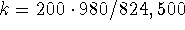dynes/cm; the model is 200 x'' + 24,500 x = 0, x(0) = 0, x'(0) = -6.

B
Use characteristic equations: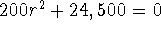;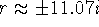. Hence, a general solution is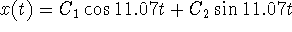. The initial conditions force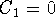, C2 = -.06/11.07 = -.00542 . Hence,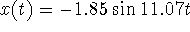.
C
To find the period, solve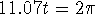to find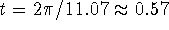s.
D
The maximum displacement occurs inwhen the sine function is. Hence, the maximum displacement (or amplitude of the motion) is 1.85 cm.

2
A
Use undetermined coefficients. First guess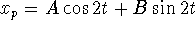. Because these functions are solutions of the homogeneous equation, the correct guess is. Substitute and collect coefficients of sine and cosine to find A = 0, B = 1/4. Hence,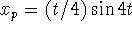.
B
Using the particular solution just found and the given homogeneous solutions,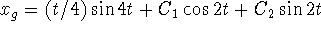.
C
Because of the leading factor of t in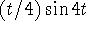, the solution grows without bound. The system is being forced at its resonant frequency.

3
A
r = -4, 2
B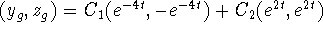.
C
Choose,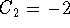.

4
A
Write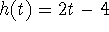.

B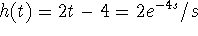C
Let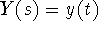. Thenand•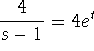. Using partial fractions, we findThen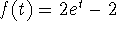,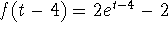, and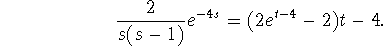Hence, the solution is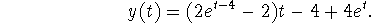• ```

© 1996 by Will Brother.
All rights Reserved. File last modified on December 6, 1996.

```